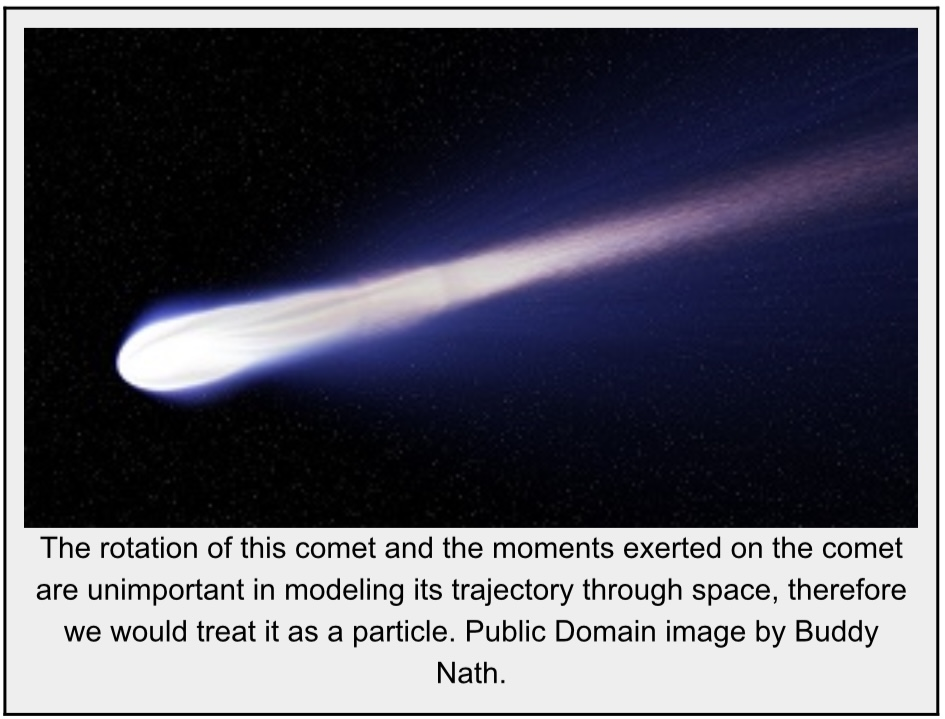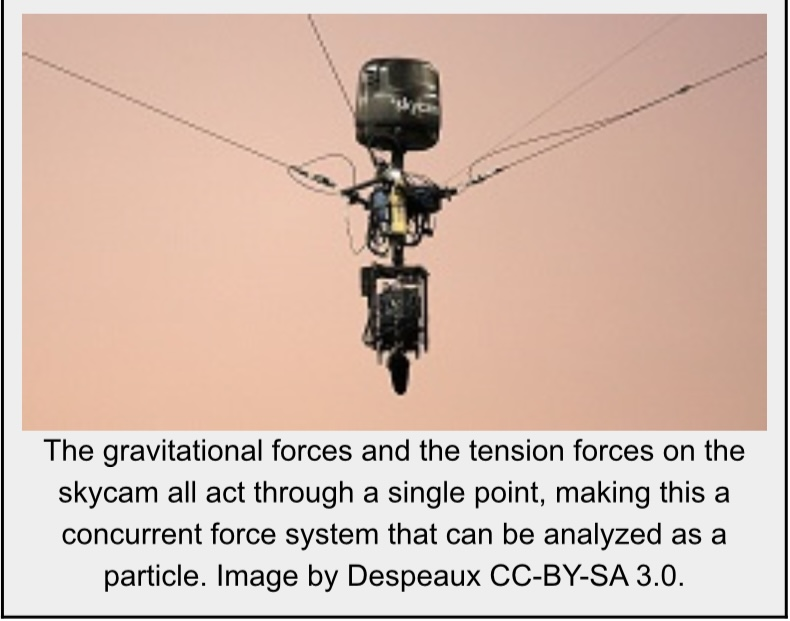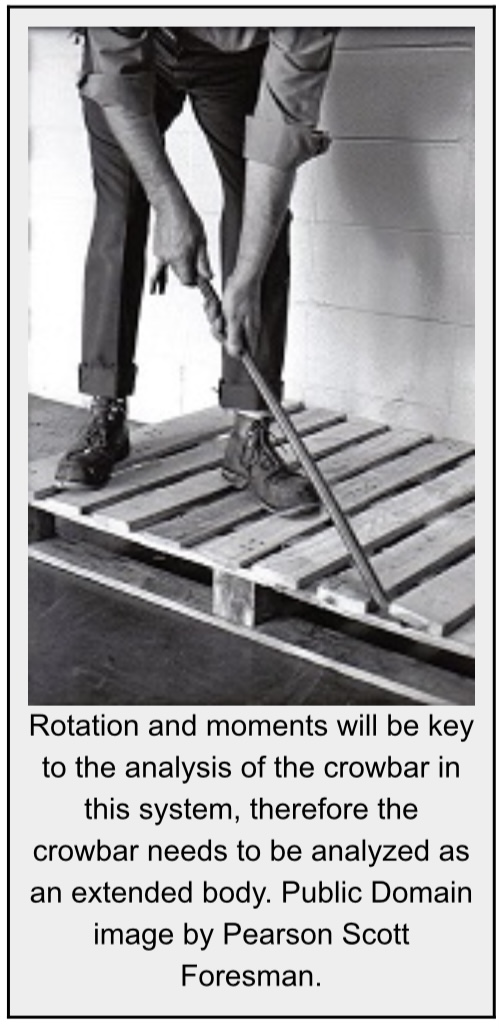Chapter 2: Particles

# 2.1 Particle & Rigid Body

This may seem like a simple concept, but it is important to know the difference between particles and rigid bodies, because it will change the type of analysis you perform.

Particles are bodies where all the mass is concentrated at a single point in space. Particle analysis will only have to take into account the forces acting on the body and translational motion because rotation is not considered for particles.

Rigid bodies on the other hand have mass that is distributed throughout a finite volume. Rigid body analysis is more complex and also has to take into account moments and rotational motions. In actuality, no bodies are truly particles, but some bodies can be approximated as particles to simplify analysis. Bodies are often assumed to be particles if the rotational motions are negligible when compared to the translational motions, or in systems where there is no moment exerted on the body such as a concurrent force system.Source: Engineering Mechanics, Jacob Moore, et al. http://www.oercommons.org/courses/mechanics-map-open-mechanics-textbook/view

Particles are typically part of a larger scale, such as a sky diver falling through the sky, or a football flying through the air. Rigid body analyses are required when the length or size of the object must be considered, such as if you need to calculate the torque from turning a bolt with a wrench, or if there is rotation, such as the bolt that is being turned.

One way to think of it is that particles have mass, whereas rigid bodies have mass and shape. We make an assumption that neither particles nor rigid bodies deform (change shape). Note: we say particles don’t deform even though we are already assuming that the shape of particles is negligible.

In baseball, if you want to consider how far the ball travels, that would be a particle analysis because the speed is much greater than the size of the ball. A rigid body analysis would be how the bat swings to hit the ball, because the length of the bat would change how far the ball travels. A rigid body analysis could be to calculate the spin on the ball as it flies through the air (if you focus on how it is rotating).

You would have done particle analyses in your high school physics classes. Starting in chapter 3, we’ll expand on these concepts to include rigid bodies and bring shape and size into the problem.

Key Takeaways

Basically: Particles have non-deforming mass & rigid bodies have non-deforming mass with shape & size. Rigid body analyses are required when length or size of the object much be considered, including rotation and torque. Particle analyses are for a grander scale where the object is small in comparison to the distance or speed.

Application: A particle analysis would be an airplane as it flies at a high speed through the air. A rigid body analysis would be if you analyze how the plane is rotating in order to turn, or to consider the size of the wheels as it is taxing on the runway or the size of the wings to keep it in the air.

Looking ahead: Chapter 2 concerns particle analyses and Chapters 3 – 7 focus on rigid body analyses.